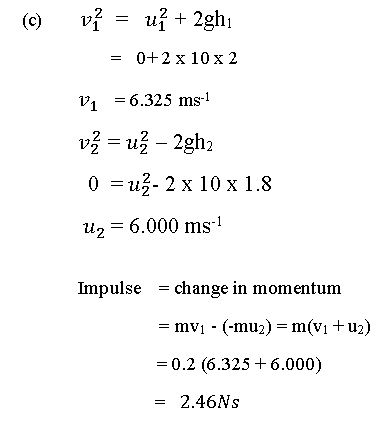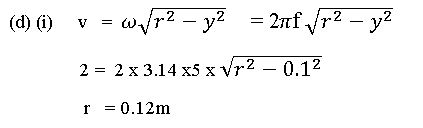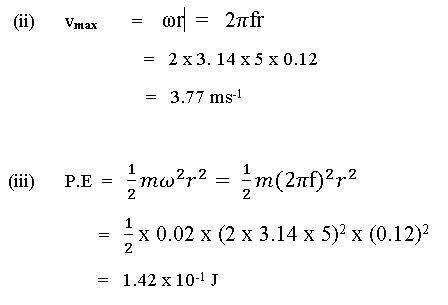Question 8

(a) Explain the term net force

(b) Define the principle of conservation of linear momentum and state one example of it

(c) A ball of mass 200 g released from a height of 2.0 m hits a horizontal floor and rebounds to a height of 1.8 m. Calculate the impulse received by the floor. (g = 10 ms-2)

(d) A body of mass 20 g performs a simple harmonic motion at a frequency of 5 Hz.

At a distance of 10 cm from the mean position, its velocity is 200 cms-1. Calculate its:

i. Maximum displacement from the mean position;

ii. Maximum velocity;

iii. Maximum potential energy (g = 10 ms-2; π = 3.14)

Observation

Part (a) This was the most popular choice of candidates but the recorded performance was low. Candidates failed to acknowledge that Net force causes motion/acceleration.

Part (b) Many candidates attempted this question. Almost all candidates failed to give a correct statement and example to deserve full marks in place of outright zero. They failed to accord the necessary sense of direction to a conserve momentum when it is a linear momentum’

Performance was low.

Part (c) Many candidates did not attempt this question. This question exposed the inadequacies of the candidate’s knowledge of “Impulse”. Some candidates added both the times of the upward and downward motion and computed the weight of the ball to obtain the Impulse. Although, the value obtained agreed with the expected result but the approach was totally unacceptable.

Performance was low.

Part (d) Majority of the candidates did not attempt this question. Some candidates right from the d(i) got the solutions wrong which extended to other sub questions (ii and iii). Also, the candidates’ inability to use appropriate units in their calculation and correct equation for the simple harmonic motion contributed to the average performance.
Performance was average.

8.   (a)   Net force is the effective force resulting from the actions of a system of forces
on a body.
OR
Net force is an unbalanced force that produces acceleration of a body.
(b)      In an isolated/closed system of colliding bodies the total linear momentum in a  fixed direction remains unchanged/constant.
(Accept any other valid statement)
e.g    rocket propulsion;
recoil of gun;
colliding trolleys.

Any correct 1 x 1 mark